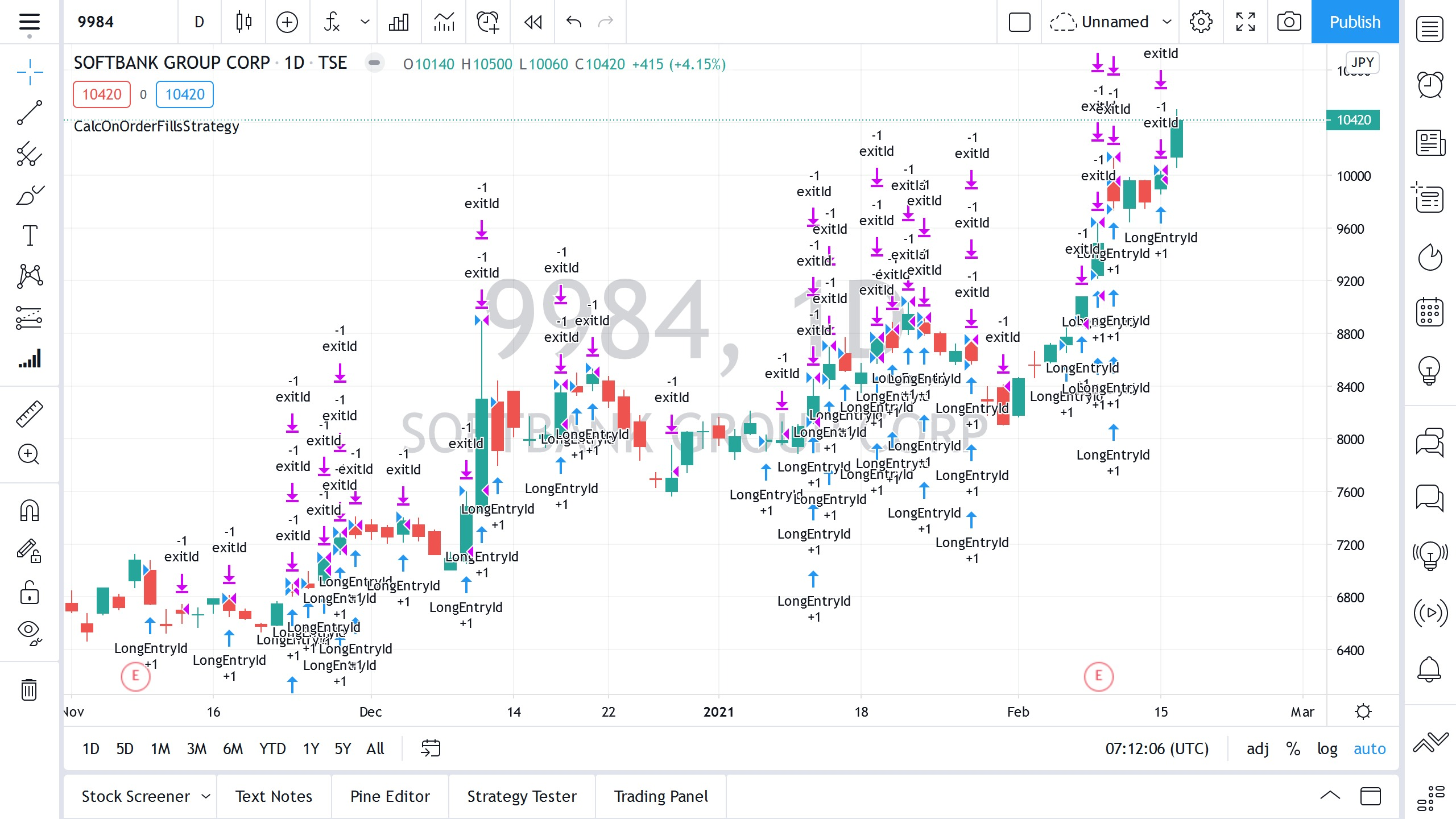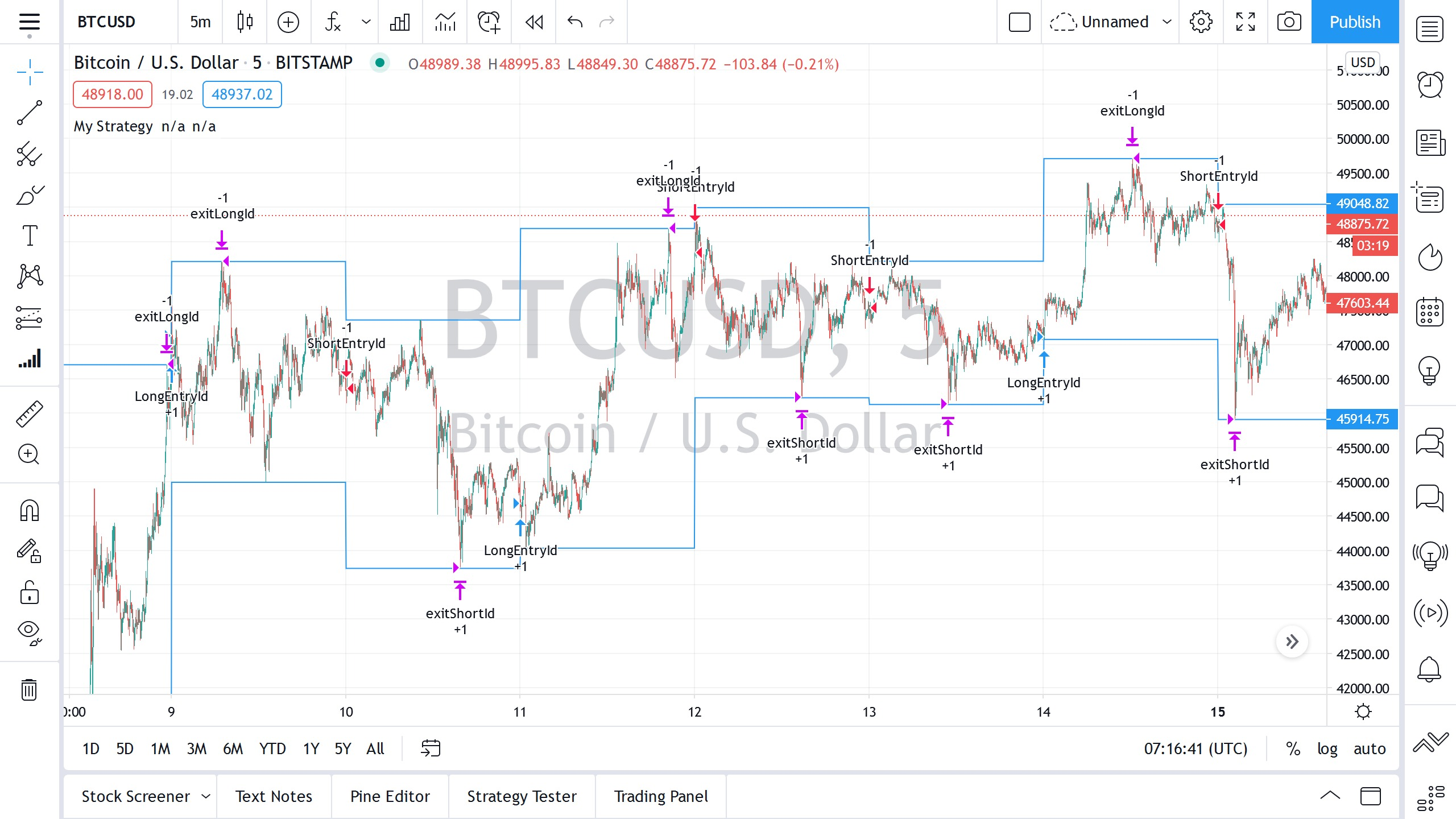# 通过窥视未来，策略产生不切实际的良好结果

``````//@version=4
strategy("My Strategy", overlay=true)
if close < close    strategy.entry("ShortEntryId", strategy.short)
strategy.close("ShortEntryId", when = close > close)if close > close    strategy.entry("LongEntryId", strategy.long)
strategy.close("LongEntryId", when = close < close)``````
JavaScript``````//@version=4
strategy("CalcOnOrderFillsStrategy", overlay=true, calc_on_order_fills=true)// a variable is used to prevent double entry on the same bar
var lastTimeEntry = 0
longCondition = close > sma(close, 14)  and lastTimeEntry != time
if longCondition    strategy.entry("LongEntryId", strategy.long)
strategy.exit("exitId", "LongEntryId", limit=high)
lastTimeEntry := time``````
JavaScriptPine中的安全功能允许您从其他交易品种和/或时间周期中请求数据。根据执行方式的不同，这可能使该策略可以接收来自未来的数据：例如，如果有请求日K线的收盘价或最高价，当回测该策略时，则可以在一天的开盘时就知道这些值。

``````//@version=4
strategy("My Strategy", overlay=true)
dayLow = security(syminfo.tickerid, "1D", low, lookahead=barmerge.lookahead_on)// entry at first bar of a day
if time == dayStart    // distance to daily high is further, so we can earn moreif abs(open - dayHigh) > abs(open - dayLow)        strategy.entry("LongEntryId", strategy.long)        strategy.exit("exitLongId", "LongEntryId", limit=dayHigh)else        strategy.entry("ShortEntryId", strategy.short)        strategy.exit("exitShortId", "ShortEntryId", limit=dayLow)plot(dayHigh)
plot(dayLow)``````
JavaScript